## Comparison of Conductor Material in Overhead System:

In comparing the relative amounts of Conductor Material in Overhead System necessary for different systems of transmis­sion, similar conditions will be assumed in each case viz.,

• same power (P watts) transmitted by each system.
• the distance (1 metres) over which power is transmitted remains the same.
• the line losses (W watts) are the same in each case.
• the maximum voltage between any conductor and earth (Vm) is the same in each case.

### 1.Two-Wire D.C. system with one conductor earthed:

In the 2-wire d.c. system, one is the outgoing or positive wire and the other is the return or negative wire as shown in Fig. 7.4. The load is connected between the two wires.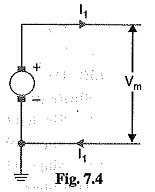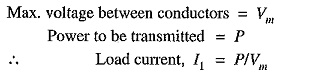If R1 is the resistance of each line Conductor Material in Overhead System, then,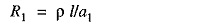where a1 is the area of X-section of the conductor.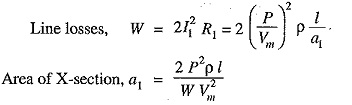Volume of conductor material requited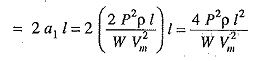It is a usual practice to make this system as the basis fo  comparison with other systems. There­fore, volume of conductor material required in this system shall be taken as the basic quantity i.e.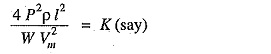### 2.Two-Wire D.C. system with mid-point earthed:

Fig. 7.5 shows the two-wire d.c. system with mid-point earthed. The maximum voltage between any conductor and earth is Vm so that maximum voltage between conductors is 2Vm.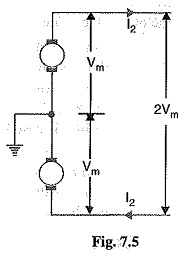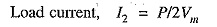Let a2 be the area of X-section of the conductor.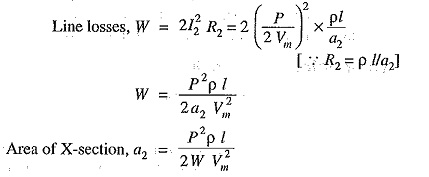Volume of conductor material required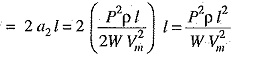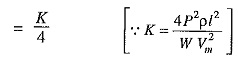Hence, the volume of Conductor Material in Overhead System required in this system is one-fourth of that required in a two-wire d.c. system with one conduc-tor earthed.

### 3.Three-wire d.c. system:

In a 3-wire d.c. system, there are two outers and a middle or neutral wire which is earthed at the genera-tor end as shown in Fig. 7.6. If the load is balanced, the current in the neutral wire is zero. Assuming balanced loads,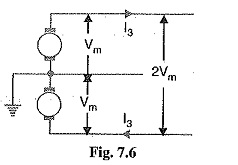Let a3 be the area of X-section of each outer wire.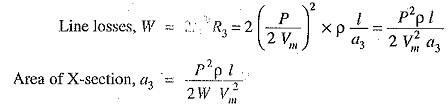Assuming the area of X-section of neutral wire to be half that of the outer wire,

Volume of conductor material required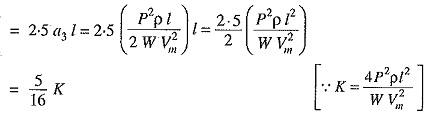Hence the volume of Conductor Material in Overhead System required in this system is 5/16th of what is required for a 2-wire d.c. system with one conductor earthed.

### 4. Single phase 2-wire a.c. system with one conductor earthed:

Fig. 7.7 shows a single phase 2-wire a.c. system with one conductor earthed. The maximum voltage between conductors is Vm so that r. m.s. value of voltage between them is Vm/√2. Assuming the load power factor to be cos Φ,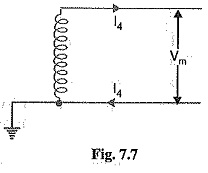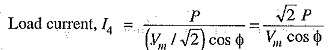Let a4 be the area of X-section of the conductor.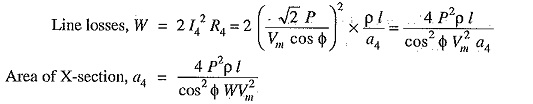Volume of conductor material required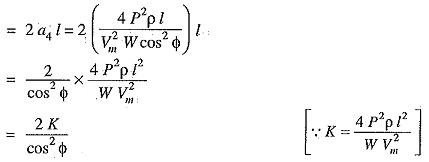Hence, the volume of Conductor Material in Overhead System required in this system is 2/cos2Φ times that of 2-wire d.c. system with the one conductor earthed.

### 5.Single phase 2-wire system with mid-point earthed:

Fig. 7.8 shows a single phase mc. system with mid-point earthed. The two wires possess equal and opposite voltages to earth (i.e., Vm). Therefore, the maximum voltage between the two wires is 2V,„. The r.m.s. value of voltage between conductors is = 2Vm/√2=√2Vm.. Assuming the power factor of the load to be cos Φ,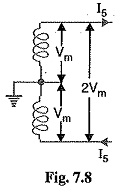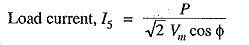Let a5 be the area of X-section of the conductor.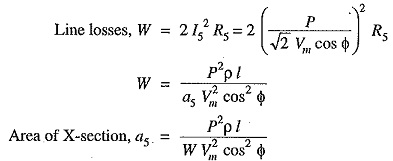Volume of conductor material required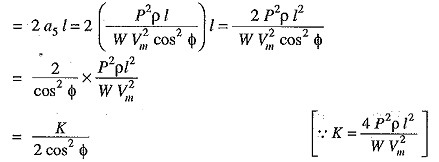Hence the volume of conductor material required in this system is 1/2cos2Φ times that of 2-wire d.c. system with one conductor earthed.

### 6.Single phase, 3-wire system:

The single phase 3-wire system is identical in principle with 3-wire d.c. system. The system consists of two outers and neutral wire taken from the mid-point of the phase wind­ing as shown in Fig. 7.9. If the load is balanced, the current through the neutral wire is zero. Assuming balanced load,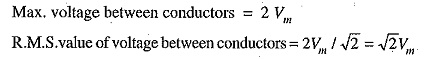If the p.f of the load is cos Φ, then,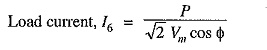Let a6 be the area of X-section of each outer conductor.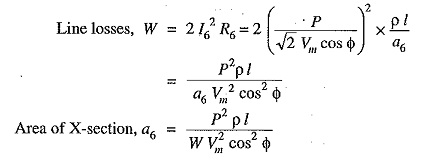Assuming the area of X-section of neutral wire to be half that of the outer wire,

Volume of conductor material required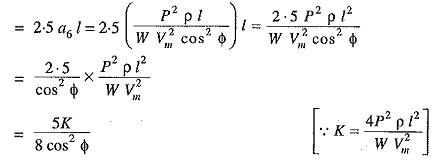Hence, the volume of Conductor Material in Overhead System required in this system is 5/8 cos2 Φ times that required in a 2-wire d.c. system with one conductor earthed.

### 7.Two phase, 4-wire a.c. system:

As shown in Fig. 7.10, the four wires are taken from the ends of the two-phase windings and the mid-points of the two windings are connected together. This system can be considered as two independent single phase systems, each trans­mitting one half of the total power.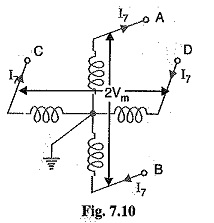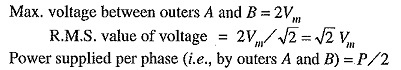Assuming p.f. of the load to be cos Φ,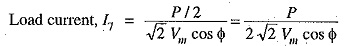Let a7 be the area of X-section of one conductor.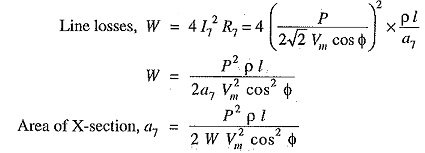Volume of conductor material required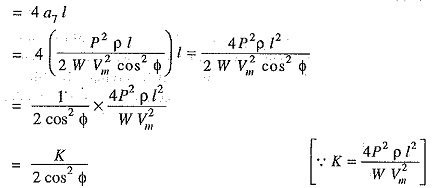Hence, the volume of Conductor Material in Overhead System required for this system is 1/2cos2Φ times that of 2 wire d.c. system with one conductor earthed.

### 8.Two-phase, 3-wire system:

Fig. 7.11 shows two-phase, 3-wire a.c. system. The third or neutral wire is taken from the junction of two-phase windings whose voltages are in quadrature with each other. Obviously, each phase transmits one half of the total power. The R.M.S. voltage between outgoing conductor and neutral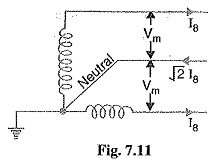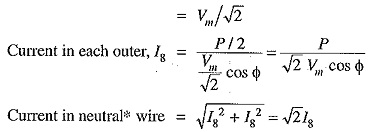Assuming the current density to be constant, the area of X-section of the neutral wire will be √2 times that of either of the outers.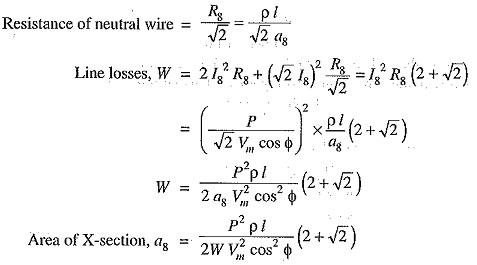Volume of conductor material required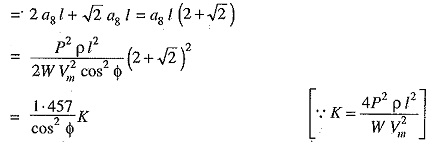Hence, the volume of conductor material required for this system is 1.457/cos2Φ times that of 2-wire d.c. system with one conductor earthed.

### 9.3-Phase, 3-wire system:

This system is almost univer­sally adopted for transmission of electric power. The 3-phase, 3- wire system may be star connected or delta connected. Fig. 7.12 shows 3-phase, 3-wire start connected system. The neutral point N is earthed.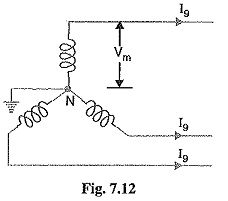Volume of conductor material required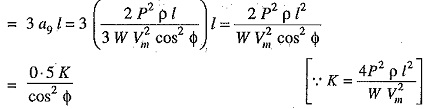Hence, the volume of conductor material required for this sys­tem is 0.5/cos2Φ times that required for 2-wire d.c. system with one conductor earthed.

### 10.3-phase, 4-wire system:

In this case, 4th or neutral wire is taken from the neutral point as shown in Fig. 7.13. The area of X-section of the neutral wire is generally one-half that of the line conductor. If the loads are balanced, then current through the neu­tral wire is zero. Assuming balanced loads and pl. of the load, as cos Φ,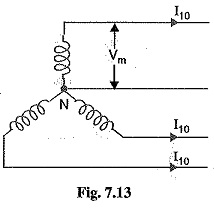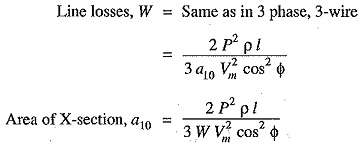As the area of X-section of neutral wire is one-half that of any line conductor,

Volume of conductor material required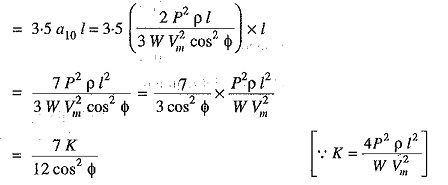Hence, the volume of Conductor Material in Overhead System required for this system is 7/12cos2Φ times that required for 2-wire d.c. system with one conductor earthed.

Scroll to Top